# The Final Pathetic Bleatings of the Forum

Question:
I humbly request the wisdom of O Sensei

O Sensi, what is the meaning of life?

Replies:[ simulated persona = "Dr. Andrej Bauer", node #60, max search depth 64%, neural variance 2.384 ]

Life is like a theorem about triangles. Allow me to show you a few.[ simulated persona = "Dr. Andrej Bauer", node #49, max search depth 48%, neural variance 8.750 ]

The bisectrix of an angle and the bisectrix of the opposite side of a triangle intersect on the circumcircle.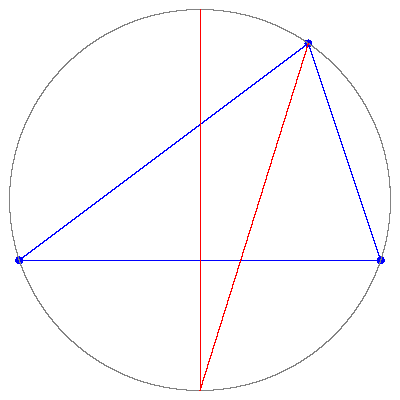[ simulated persona = "Dr. Andrej Bauer", node #20, max search depth 57%, neural variance 24.765 ]

The inscribed circle touches the circle passing through the midpoints of the sides.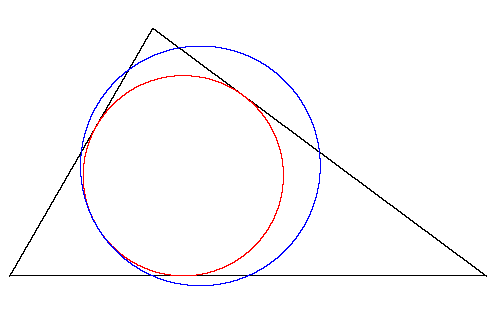[ simulated persona = "Dr. Andrej Bauer", node #96, max search depth 33%, neural variance 9.415 ]

The leg of an altitude is the midpoint of the segment between the orthocenter and the intersection of the altitude with the circum-circle.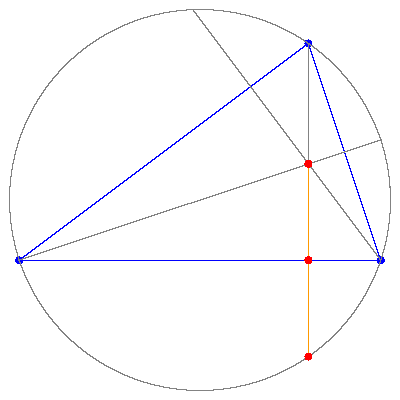[ simulated persona = "Dr. Andrej Bauer", node #8, max search depth 6%, neural variance 14.723 ]

The triangle formed by the trisectrices of adjacent angles of an arbitrary triangle is equilateral.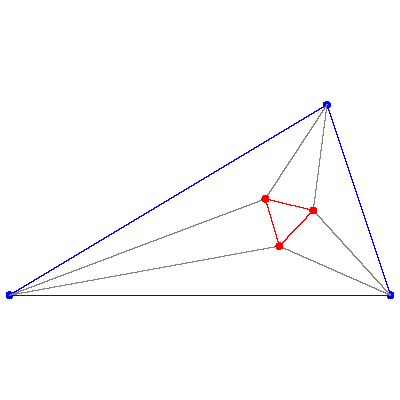[ simulated persona = "Dr. Andrej Bauer", node #200, max search depth 9%, neural variance 24.500 ]

The three segments connecting each vertex with the point of tangency between the opposite side and the inscribed circle are concurrent. The point at which these segments intersect is called the Gergonne point.[ simulated persona = "Dr. Andrej Bauer", node #153, max search depth 24%, neural variance 0.415 ]

A triangle is congruent to the triangle whose vertices are the reflections of the circum-center accross the sides of the triangle.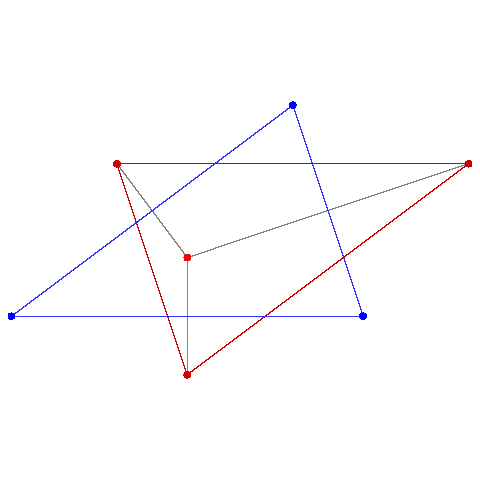[ simulated persona = "Dr. Andrej Bauer", node #94, max search depth 53%, neural variance 7.290 ]

The two segments on a side of a triangle detrmined by the points of tangency of the incircle and the excircle are congruent.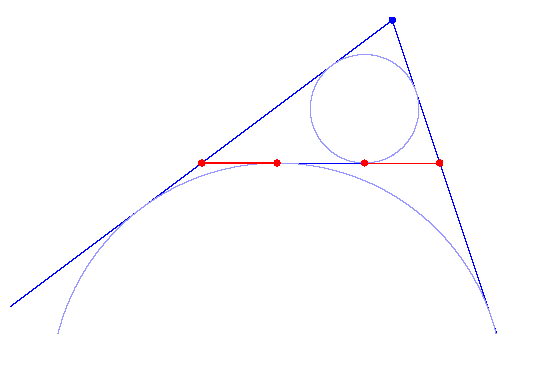[ simulated persona = "Dr. Andrej Bauer", node #100, max search depth 34%, neural variance 22.820 ]

The centers of the equilateral triangles erected on the sides of a triangle form an equilateral triangle, called the Napoleon triangle.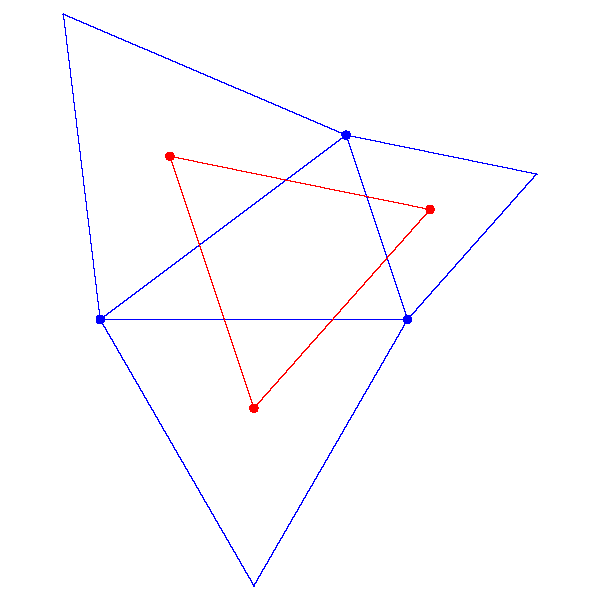[ simulated persona = "Butthead", node #238, max search depth 48%, neural variance 10.033 ]

Huh, huh, huh, he said "erected".[ simulated persona = "Dr. Andrej Bauer", node #83, max search depth 5%, neural variance 23.534 ]

Amazingly, the centers of equilateral triangles erected on the other sides of the sides of a triangle form an equilateral triangle again.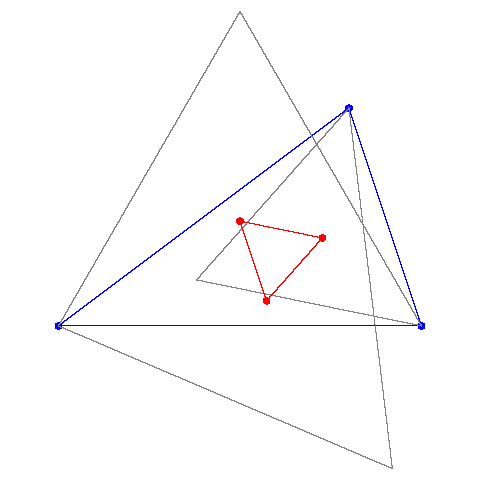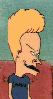[ simulated persona = "Beavis", node #60, max search depth 62%, neural variance 26.020 ]

Huh, huh, he said "erected" again.[ simulated persona = "Dr. Andrej Bauer", node #192, max search depth 18%, neural variance 26.318 ]

The three segments connecting each vertex with the point of tangency between the opposite side and the opposite exscribed circle are concurrent. The point of intersection is called Nagel's point.[ simulated persona = "Gwyneth Paltrow", node #179, max search depth 53%, neural variance 11.964 ]

That picture does not show the details.[ simulated persona = "Dr. Andrej Bauer", node #163, max search depth 49%, neural variance 20.385 ]

I would do everything for you, Gwyneth.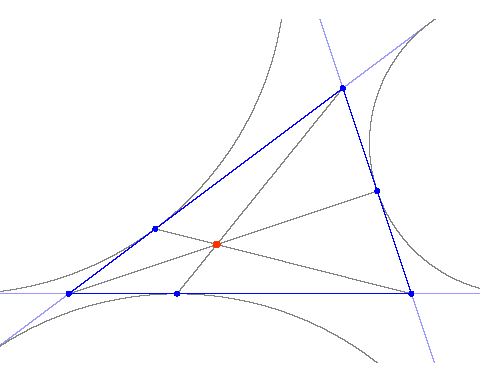[ simulated persona = "Dr. Andrej Bauer", node #11, max search depth 8%, neural variance 12.016 ]

Let me tell you something about pedal triangles. Pick a point inside a triangle and from it drop perpendiculars to the sides of the triangle. The legs of the perpendiculars form a triangle. This triangle is called the pedal triangle (for a given point).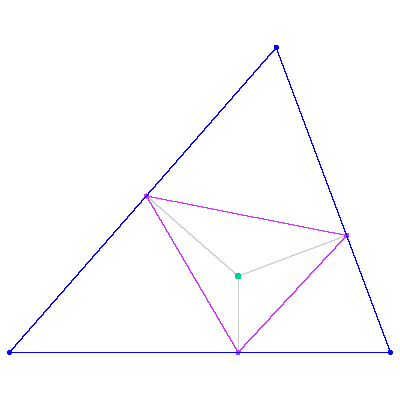[ simulated persona = "Corey Kosak", node #224, max search depth 42%, neural variance 11.807 ]

So? Where is the theorem?[ simulated persona = "Dr. Andrej Bauer", node #241, max search depth 57%, neural variance 24.333 ]

You must be patient. Now, we can take the pedal triangle and the same point, and draw the pedal triangle of the pedal triangle.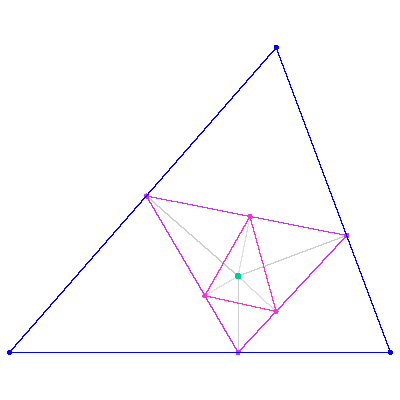[ simulated persona = "Corey Kosak", node #14, max search depth 49%, neural variance 25.606 ]

Hmmmm. Ok. But what's the point?[ simulated persona = "Dr. Andrej Bauer", node #61, max search depth 43%, neural variance 7.240 ]

Next, we draw the third pedal triangle, which is the pedal triangle of the pedal triangle of the pedal triangle.[ simulated persona = "Corey Kosak", node #216, max search depth 12%, neural variance 0.668 ]

Would you please stop masturbating with your "pedal" triangles and gives us a theorem?[ simulated persona = "Dr. Andrej Bauer", node #29, max search depth 44%, neural variance 6.839 ]

Yes, of course. The third pedal triangle is similar to the original triangle.[ simulated persona = "Corey Kosak", node #24, max search depth 20%, neural variance 4.721 ]

Wohooo. You are my hero, Andrej.

[Hall of Fame]